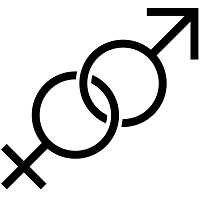### Online | home Maths, Calculus teacher needed in Hyderabad

• Kalyan Nagar Phase 1, Hyderabad, Telangana, India
• Posted : Sep 12
•Level : Intermediate
•Requires : Part Time
• Posted by : Arvind Vinjamoori (Student )
• Phone verified +91-**********
•Gender Preference : None
• Available online
• Available for home tutoring
• Can not travel

Calculus:
• Functions and Limits
• Differentiation and Integration
• Complex Numbers
• Functions of Several Variables
Differential Equations:
• First Order Equations
• Second and Higher Order Equations
Probability:
• Probability Distribution Function
• Cumulative Distribution
• Expectation Algebra
• Key Discrete and Continuous Distributions Including the Normal Distribution
• Central Limit Theorem
Statistics:
• General Summary Statistics
• Maximum Likelihood Estimator
• Regression and Correlation
Linear Algebra:
• Matrices and Vectors
• Systems of Linear Equations
• Eigenvalues and Eigenvectors